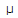# Electronics - Capacitors - Discussion

### Discussion :: Capacitors - General Questions (Q.No.4)

4.What is the reactive power in the given circuit?

 [A]. 0 VAR [B]. 691VAR [C]. 44.23 mVAR [D]. 1.45 kVAR

Explanation:

No answer description available for this question.

 Lalit Prasad said: (Dec 20, 2010) How the problem can be solved?

 Ananya said: (Jan 8, 2011) How it can be done?

 Veer said: (Feb 20, 2011) Find out Xc=1/2*3.142*f*c then P=V^2/Xc

 Shilpa said: (Mar 27, 2011) Not so clear...

 Gunda said: (Apr 23, 2011) Ya the above example is not that clear.

 Deepika said: (May 18, 2011) What is ment by reactive power? and also explaine the above problem.

 Nagashree said: (May 23, 2011) Reactive power is the amount power that is sent back to the source from load.

 Rajasree said: (Jun 25, 2011) We know that, p=v^2/R Here R is the reactance of capacitor which denoted by Xc Xc=1/(2*pi*f*c) Xc=1446.8631 ohm P=V^2/Xc P=64/1446.8631 P=44.233 mw

 Niin Mukesh said: (Aug 23, 2011) p=v^2/R and we know, Xc=1/(2*pi*f*c) Xc=1446.8631 ohm P=V^2/Xc P=64/1446.8631 P=44.233 mw

 Sindhu said: (Dec 3, 2011) v^ and pi and f means am not understand

 Divz said: (Dec 12, 2011) v^2 - v to the power 2 pi- const f= freq of power source

 T.B.Tandel said: (May 4, 2012) But here given voltage r.m.s value.

 Prashant said: (May 21, 2012) Xc=1/(2(pi)fc) f=5k c=0.022micro Xc=1446.86 P=V^2/Xc=8^2/1446.86 P=0.04423VAR ~ 44.23m VAR

 Jithin Kk said: (Jun 2, 2012) So what about the rms value of voltage.

 Engr. Shahbul said: (Jun 5, 2012) Xc=1000/2*3.14*5*.022=1447.597ohm. P=v2/Xc=8*8/1447.597=0.0442112w, =44.21mW.

 Salman said: (Jan 26, 2013) Xc=1/wc. w=2*pi*f. Reactive power Q=Vrms/Xc.

 Brajesh Singh said: (Apr 3, 2013) Reactive power = V(rms)*I(rms)*sin(angle b/w current and voltage). Angle = 90 (bcoz capacitor here). So P(reactive )= 8*(8*2*3.14*50k*.022micro). P = 44.21 mW.

 San said: (Apr 9, 2013) R IS THE REACTANCE OF THE CAPACITANCE Xc. XC = 1/2*3.14*F*C. = 1/6.28*5000*0.022*10^-6. = 1/31400*0.022*10^-6. = 1447.59. P = R^2/Xc = 64/1447.59 = 0.0442W = 44.21mW.

 Makkk said: (Apr 29, 2013) What is the reason behind sending the power to source back?

 Krishna said: (Aug 1, 2013) Reason behind sending back is because there is no dissipative path (load). In ideal case capacitor cannot dissipate. Apparent power is pure resistive power whereas reactive power comes into picture because of capacitive reactance in the circuit. P = v^2/R but now it is v^2/Z where z = R+Xc (Xc=1/2*pi*f*c)here R = 0 ideal case. Xc=1/2*pi*f*c. = 1/6.28*5000*0.022*10^-6. = 1/31400*0.022*10^-6. = 1447.59. P = R^2/Xc = 64/1447.59 = 0.0442W = 44.21mW.

 Shashi said: (Nov 19, 2013) Power reactive capacitance's formula is "P = V^2/Xc". but now we don't know What is the Xc(Capacitance reactance)so first we should find Xc. Xc = 1/2*pi*F*C. Xc = 1446.86 ohm. P = V^2/Xc. = 8^2/1446.86. = 64/1446.86. = 0.4423 VAR. = 44.23 mVAR.

 Gomathi said: (Jan 16, 2014) Why they use the unit VAR (IN ANS). If it's one of the unit of mean where we use?

 Rachna said: (Feb 7, 2014) How the problem can be solve?

 Kiran said: (May 1, 2014) VAR - Volt Ampere Reactance (Units of reactive power).

 Siva said: (Dec 18, 2014) In our home we see 230 v whether it is RMRS value or anything.

 Savi said: (May 30, 2015) I didn't understand please tell me easy way.

 Pavan Kumar said: (Jul 16, 2015) Reactive Power is the power which is lost in the circuit and hence not delivered to the Load.

 Harika said: (Oct 12, 2015) Please can anyone explain the derivation of P=V^2/Xc?

 Adarsh said: (Jan 17, 2016) Yes as we know that: P = v^2/R. Where R is Reactant denoted by Xc. Xc = 1/2*pi*f**c. Where pi-constant, f-frequency & c-capacitance.

 Yashu said: (Apr 27, 2016) What is the value of Pi?

 Chandra said: (May 7, 2016) @Yashu. 22/7 = 3.142.

 Md Asif said: (Jun 21, 2016) P = vi. P = v^2/R. Where r = capacitive load . Xc = 1/2 * pi * f * c. Xc = 1446.86 ohm. P = 64/1446.86. P = 44.21mw.

 Kiran V said: (Nov 10, 2016) Xc = 1/2 * π * f * c. Xc = 144.75 ohm. P = 64/144.75. P = 0.4421 Var.

 Asha said: (Jul 17, 2017) Here RMS value is given and everyone is taking it directly. Why we are not converting it into max value?

 Rasika Chougale said: (Sep 17, 2019) Good discussion, thanks everyone for explaining it.